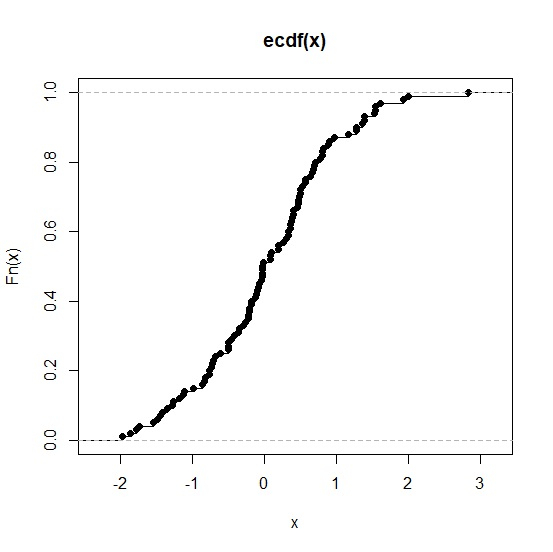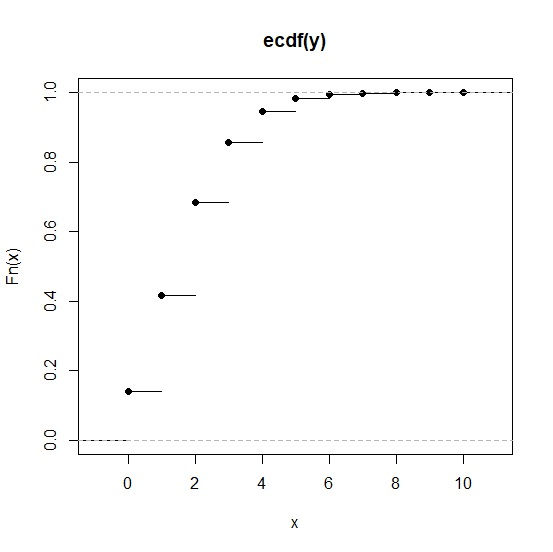# How to create a plot of empirical distribution in R?

The empirical distribution can be found by using the function ecdf and if we want to create a plot of empirical distribution then plot function will be used.

For example, if we have a vector called X then plot of empirical distribution can be created by using the below command −

plot(ecdf(X))

## Example 1

To create a plot of empirical distribution in R, use the code given below −

x<-rnorm(100)
plot(ecdf(x))

## Output

If you execute the above given code, it generates the following output −## Example 2

To create a plot of empirical distribution in R, use the code given below −

y<-rpois(10000,2)
plot(ecdf(y))

## Output

If you execute the above given code, it generates the following output −